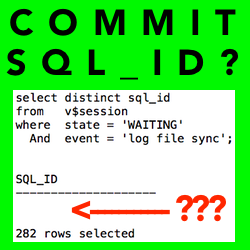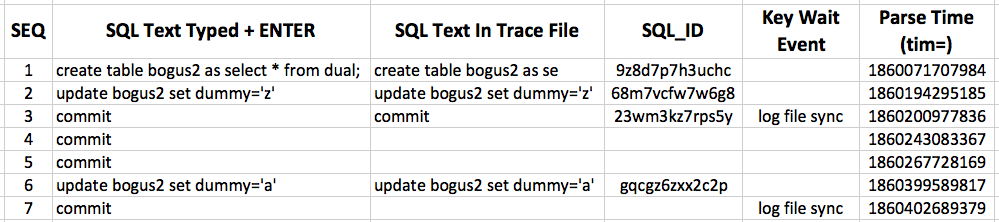## Does An Oracle Commit Always Have A Blank, Empty Or Null SQL_ID?

Posted on 30-June-2015 by Craig Shallahamer, craig@orapub.comIf the SQL_ID is empty, can I infer the session is committing? If not, then how do I know what the session is doing? And, how do I improve performance of an active session if I don't know what it's doing?

It is common for an active Oracle Database session to have a blank, empty or null SQL_ID. And, this blank SQL_ID could be the top SQL_ID based on total wait time. Furthermore, the associated Oracle Database wait event could be a log file sync.

But is the associated SQL_ID for a log file sync wait ALWAYS blank? Based on my previous post and the image shown here, it would be easy to infer this.

### The Situation

In my last article I showed that 100% of the active sessions waiting on a log file sync had a blank SQL_ID. Perhaps your system is similar. Here is a simple SQL script you can run to check and see.

``````select sql_id,
round(
100*count(*)/(
select count(*) value
from   v\$active_session_history
where  session_state = 'WAITING'
and  event         = 'log file sync')
,2) pct_waiting
from   v\$active_session_history
where  session_state = 'WAITING'
and  event = 'log file sync'
group by sql_id
order by 2 desc;
``````

I personally have never seen a committing session without some associated log file sync wait time. Never. This does not prove you will always see log file sync time during a commit, but I have never personally seen or recognized it.

### My Honest Questions

With all this in mind, consider this.

• When a commit is issued, will there always be a log file sync wait event?
• Does a commit statement have an associated SQL_ID? Is it empty? Is it always empty?
• When an active Oracle session is committing and posting a log file sync wait event, can there be an associated SQL_ID?

I am going to try to demonstrate that a committing process can indeed have a non-blank SQL_ID. If I succeed, then I know that while a blank SQL_ID can be related to an active Oracle session in the middle of a commit, there can be commits occurring that do in fact have a SQL_ID.

The remainder of this post is focused on answering these questions; How I setup the experiment, analyzed the results, and attempt to summarize the results in a meaningful and useful way. Read on!

### The Setup

In my experiment, I only use one Oracle Database session. This session will enable a level 12 trace of itself using dbms_monitor, run some SQL and then exit. Then I will make a few comments about what we find in the trace file, summarize and be done with it.

At this point, the session is connected to Oracle Database 12c. Here's the SQL.

``````SQL> SELECT VALUE FROM V\$DIAG_INFO WHERE NAME = 'Default Trace File';

VALUE
---------------------------------------------------------------------
/home/oracle/base/diag/rdbms/prod40/prod40/trace/prod40_ora_12257.trc

SQL> select sid, serial# from v\$session where sid = SYS_CONTEXT('USERENV','SID');

SID    SERIAL#
---------- ----------
401      41083

SQL> exec dbms_monitor.session_trace_enable(401,41083,waits=>TRUE);

PL/SQL procedure successfully completed.

SQL> create table bogus2 as select * from dual;

Table created.

SQL> update bogus2 set dummy='z';

1 row updated.

SQL> commit;

Commit complete.

SQL> commit;

Commit complete.

SQL> commit;

Commit complete.

SQL> update bogus2 set dummy='a';

1 row updated.

SQL> commit;

Commit complete.

SQL> exit
Disconnected from Oracle Database 12c Enterprise Edition Release 12.1.0.2.0 - 64bit Production
With the Partitioning, OLAP, Advanced Analytics and Real Application Testing options
[oracle@sixcore ~]\$
``````

The trace file did not exist until I ran the dbms_monitor session. Once that happened, then I saw this:

``````[oracle@sixcore trace]\$ ls -l /home/oracle/base/diag/rdbms/prod40/prod40/trace/prod40_ora_12257.trc
-rw-r----- 1 oracle oinstall 1362 May  1 07:23 /home/oracle/base/diag/rdbms/prod40/prod40/trace/prod40_ora_12257.trc
``````

### The Results: Detail

There is a lot of uninteresting details in the trace file. You can view the entire Oracle Database 12.1.0.2.0 level 12 trace file HERE . Below are snippets from the raw trace file. Still, there is a lot of detail you probably won't care about. So, in the next section I summarize the situation, highlighting just the key pieces.

``````=====================
PARSING IN CURSOR #139946276518960 len=41 dep=0 uid=8 oct=1 lid=8 tim=1860071707984 hv=3762106892 ad='64636958' sqlid='9z8d7p7h3uchc'
create table bogus2 as se
END OF STMT
PARSE #139946276518960:c=28000,e=28364,p=18,cr=403,cu=0,mis=1,r=0,dep=0,og=1,plh=1501550473,tim=1860071707983
EXEC #139946282300496:c=0,e=17,p=0,cr=0,cu=0,mis=0,r=0,dep=2,og=4,plh=813480514,tim=1860071708318
FETCH #139946282300496:c=0,e=18,p=0,cr=4,cu=0,mis=0,r=1,dep=2,og=4,plh=813480514,tim=1860071708361
CLOSE #139946282300496:c=0,e=4,dep=2,type=3,tim=1860071708389
EXEC #139946277694992:c=0,e=16,p=0,cr=0,cu=0,mis=0,r=0,dep=2,og=4,plh=2035254952,tim=1860071708442
WAIT #139946277694992: nam='db file sequential read' ela= 7 file#=1 block#=156 blocks=1 obj#=4 tim=1860071708484
FETCH #139946277694992:c=0,e=61,p=1,cr=5,cu=0,mis=0,r=1,dep=2,og=4,plh=2035254952,tim=1860071708524
...
=====================
...
=====================
PARSING IN CURSOR #139946276518960 len=27 dep=0 uid=8 oct=6 lid=8 tim=1860194295185 hv=3095271912 ad='609ac968' sqlid='68m7vcfw7w6g8'
update bogus2 set dummy='z'
END OF STMT
PARSE #139946276518960:c=0,e=22007,p=24,cr=193,cu=0,mis=1,r=0,dep=0,og=1,plh=2219771076,tim=1860194295184
EXEC #139946276518960:c=0,e=131,p=0,cr=3,cu=3,mis=0,r=1,dep=0,og=1,plh=2219771076,tim=1860194295377
STAT #139946276518960 id=1 cnt=0 pid=0 pos=1 obj=0 op='UPDATE  BOGUS2 (cr=3 pr=0 pw=0 time=95 us)'
STAT #139946276518960 id=2 cnt=1 pid=1 pos=1 obj=122646 op='TABLE ACCESS FULL BOGUS2 (cr=3 pr=0 pw=0 time=22 us cost=2 size=2 card=1)'
WAIT #139946276518960: nam='SQL*Net message to client' ela= 2 driver id=1650815232 #bytes=1 p3=0 obj#=-1 tim=1860194295509

*** 2015-05-01 07:29:50.400
WAIT #139946276518960: nam='SQL*Net message from client' ela= 6682038 driver id=1650815232 #bytes=1 p3=0 obj#=-1 tim=1860200977572
CLOSE #139946276518960:c=0,e=9,dep=0,type=0,tim=1860200977674
=====================
PARSING IN CURSOR #139946276518960 len=6 dep=0 uid=8 oct=44 lid=8 tim=1860200977836 hv=3480936638 ad='0' sqlid='23wm3kz7rps5y'
commit
END OF STMT
PARSE #139946276518960:c=0,e=108,p=0,cr=0,cu=0,mis=0,r=0,dep=0,og=0,plh=0,tim=1860200977835
XCTEND rlbk=0, rd_only=0, tim=1860200977905
EXEC #139946276518960:c=0,e=137,p=0,cr=0,cu=1,mis=0,r=0,dep=0,og=0,plh=0,tim=1860200978033
WAIT #139946276518960: nam='log file sync' ela= 21256 buffer#=3590 sync scn=28853279 p3=0 obj#=-1 tim=1860200999318
WAIT #139946276518960: nam='SQL*Net message to client' ela= 1 driver id=1650815232 #bytes=1 p3=0 obj#=-1 tim=1860200999395

*** 2015-05-01 07:30:32.506
WAIT #139946276518960: nam='SQL*Net message from client' ela= 42083764 driver id=1650815232 #bytes=1 p3=0 obj#=-1 tim=1860243083190
CLOSE #139946276518960:c=0,e=23,dep=0,type=1,tim=1860243083314
PARSE #139946276518960:c=0,e=8,p=0,cr=0,cu=0,mis=0,r=0,dep=0,og=0,plh=0,tim=1860243083367
XCTEND rlbk=0, rd_only=1, tim=1860243083400
EXEC #139946276518960:c=0,e=54,p=0,cr=0,cu=0,mis=0,r=0,dep=0,og=0,plh=0,tim=1860243083443
WAIT #139946276518960: nam='SQL*Net message to client' ela= 1 driver id=1650815232 #bytes=1 p3=0 obj#=-1 tim=1860243083478

*** 2015-05-01 07:30:57.151
WAIT #139946276518960: nam='SQL*Net message from client' ela= 24644523 driver id=1650815232 #bytes=1 p3=0 obj#=-1 tim=1860267728019
CLOSE #139946276518960:c=0,e=6,dep=0,type=3,tim=1860267728120
PARSE #139946276518960:c=0,e=7,p=0,cr=0,cu=0,mis=0,r=0,dep=0,og=0,plh=0,tim=1860267728169
XCTEND rlbk=0, rd_only=1, tim=1860267728202
EXEC #139946276518960:c=0,e=53,p=0,cr=0,cu=0,mis=0,r=0,dep=0,og=0,plh=0,tim=1860267728246
WAIT #139946276518960: nam='SQL*Net message to client' ela= 1 driver id=1650815232 #bytes=1 p3=0 obj#=-1 tim=1860267728274

*** 2015-05-01 07:33:09.011
WAIT #139946276518960: nam='SQL*Net message from client' ela= 131859635 driver id=1650815232 #bytes=1 p3=0 obj#=-1 tim=1860399587927
CLOSE #139946276518960:c=0,e=6,dep=0,type=3,tim=1860399588046
=====================
...
=====================
PARSING IN CURSOR #139946277880608 len=27 dep=0 uid=8 oct=6 lid=8 tim=1860399589817 hv=4224790613 ad='65a1b6d0' sqlid='gqcgz6zxx2c2p'
update bogus2 set dummy='a'
END OF STMT
PARSE #139946277880608:c=1000,e=1726,p=0,cr=7,cu=0,mis=1,r=0,dep=0,og=1,plh=2219771076,tim=1860399589816
EXEC #139946277880608:c=0,e=126,p=0,cr=3,cu=3,mis=0,r=1,dep=0,og=1,plh=2219771076,tim=1860399589999
STAT #139946277880608 id=1 cnt=0 pid=0 pos=1 obj=0 op='UPDATE  BOGUS2 (cr=3 pr=0 pw=0 time=98 us)'
STAT #139946277880608 id=2 cnt=1 pid=1 pos=1 obj=122646 op='TABLE ACCESS FULL BOGUS2 (cr=3 pr=0 pw=0 time=23 us cost=2 size=2 card=1)'
WAIT #139946277880608: nam='SQL*Net message to client' ela= 1 driver id=1650815232 #bytes=1 p3=0 obj#=-1 tim=1860399590109

*** 2015-05-01 07:33:12.112
WAIT #139946277880608: nam='SQL*Net message from client' ela= 3099088 driver id=1650815232 #bytes=1 p3=0 obj#=-1 tim=1860402689219
CLOSE #139946277880608:c=0,e=8,dep=0,type=0,tim=1860402689318
PARSE #139946276518960:c=0,e=12,p=0,cr=0,cu=0,mis=0,r=0,dep=0,og=0,plh=0,tim=1860402689379
XCTEND rlbk=0, rd_only=0, tim=1860402689410
EXEC #139946276518960:c=0,e=124,p=0,cr=0,cu=1,mis=0,r=0,dep=0,og=0,plh=0,tim=1860402689524
WAIT #139946276518960: nam='log file sync' ela= 22915 buffer#=3641 sync scn=28853373 p3=0 obj#=-1 tim=1860402712466
WAIT #139946276518960: nam='SQL*Net message to client' ela= 1 driver id=1650815232 #bytes=1 p3=0 obj#=-1 tim=1860402712539
``````

### The Results: Condensed

The table below is a condensed summary of just the pieces I want to focus on.Before I delve into what the experiment reveals, here is a short description of each table column.

SEQ is simply a sequence number for references purposes.

SQL Text Typed + ENTER is the exact text I typed at the SQL*Plus prompt and pressed enter.

SQL Text In Trace File is the exact text shown in the trace file.

SQL_ID is the statement's SQL_ID. If the cell is blank, then no SQL_ID is shown.

Key Wait Event is shown if it is of interest.

Parse Time is the "tim=" field in the trace file when the statement began or if there is no statement shown then when the parse phase "tim=" field is shown.

There is a lot that can be gleaned from the trace file... more than I want to address. My focus is on my Honest Questions that I proposed at the top of this article. So, I'm going to answer my questions based 100% on the above summarized trace file output.

• Q: When a commit is issued, will there always be a log file sync wait event?
• A: Absolutely NOT. Sequence number 4 and 5 have no associated log file sync wait event but are the result of a commit. So, there must be some associated CPU consumption time.
• Q: Does a commit statement have an associated SQL_ID? Is it empty? Is it always empty?
• A: Sometimes a commit statement has an associated SQL_ID and sometimes it does not have an associated SQL_ID.
• Q: When an active Oracle session is committing and posting a log file sync wait event, can there be an associated SQL_ID?
• A: Yes there can be an associated SQL_ID, as shown in sequence number 3. But as sequence numbers 7 shows, a commit was issued and there is an associated log file sync wait, but there is no SQL_ID.

Are you confused yet? Does this seem arbitrary? I suspect there is a perfectly logical explanation for all of this. But my objective is not to provide this perfectly logical explanation. So, let's distill this down to the bare-metal facts. Read on!

### The Results: Summarized

While I can draw a number of inferences directly from this little experiment, referencing only my experimental data;

• During a commit there can be (sequence 3) or can not be (sequence 4, 5, 7) an associated SQL_ID.
• During a commit there can be (sequence 3, 7) or can not be (sequence 4, 5) a log file sync wait event.
• The first commit after an update statement (sequence 3, 7) was associated with a log file sync wait event.

After the initial commit, subsequent commits were not associated with a log file sync wait event. (sequence 4, 5) Why not? My guess is because there is no associated wait time, only CPU consumption. It makes sense when you think about it.

And finally, the big result here is, whenever a log file sync appears, there was an associated update statement (sequence 2 and 6) followed by a commit statement (sequence 3 and 7). For me, based on this experiment and my personal experience,

whenever I see a log file sync wait event, while I don't expect to see a SQL_ID and definitely not the DML SQL_ID(s), I do know that a commit is taking place.